Courses

# Differential Equation MCQ Level - 2

## 10 Questions MCQ Test Topic wise Tests for IIT JAM Physics | Differential Equation MCQ Level - 2

Description
This mock test of Differential Equation MCQ Level - 2 for IIT JAM helps you for every IIT JAM entrance exam. This contains 10 Multiple Choice Questions for IIT JAM Differential Equation MCQ Level - 2 (mcq) to study with solutions a complete question bank. The solved questions answers in this Differential Equation MCQ Level - 2 quiz give you a good mix of easy questions and tough questions. IIT JAM students definitely take this Differential Equation MCQ Level - 2 exercise for a better result in the exam. You can find other Differential Equation MCQ Level - 2 extra questions, long questions & short questions for IIT JAM on EduRev as well by searching above.
QUESTION: 1

### (D2 - 1)y = x sin x + (1 + x2)ex, will be satisfied by which of the following functions?

Solution:

The given differential equation is,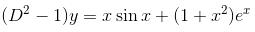The auxiliary equation is
m2 – 1 = 0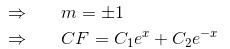or C1ex + C2ex      (as C1C2 are arbitrary constant)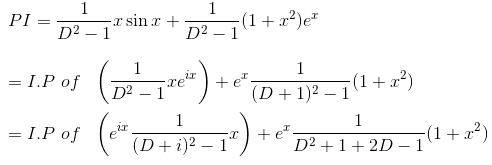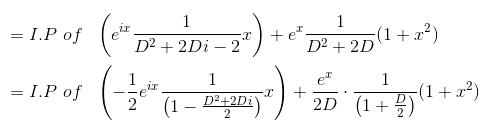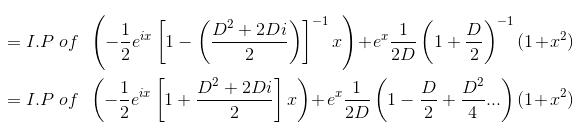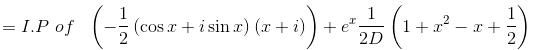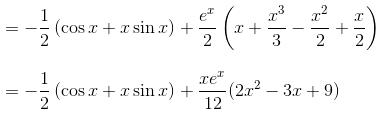Hence, y = CF + PI gives the required functions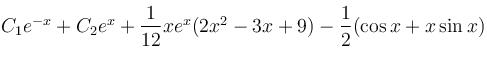QUESTION: 2

### If [(x + 1)2 f(x) – g(x)] is the particular integral of the differential equation,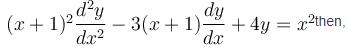Solution:

Let x + 1 = ez so that z = log (x + 1) and x = ez – 1.

The given equation becomes

[D(D – 1) – 3D + 4]y = (ez –1)2D = d/dz
or    (D2 – 4D + 4)y = e2z – 2ez + 1
The auxiliary equation is m2 – 4m + 4 = 0
⇒   (m – 2)2 = 0
∴   C.F. = [(C1 + C2z)e2z = [C1 + C2log (x + 1)] (x +1)2.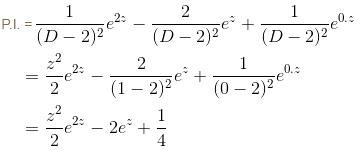∴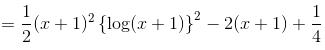The correct answer is: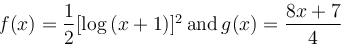QUESTION: 3

### The general solution of the differential equationwill be :

Solution:

The auxiliary equation is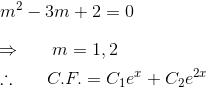Let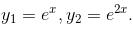Their wronskian is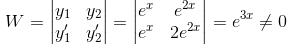P.I. = u1y1 + u2y2, where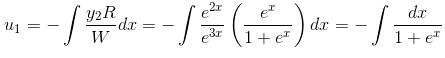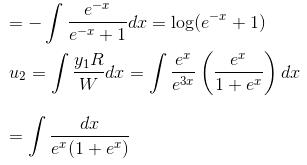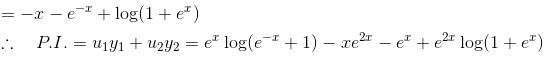Hence y = C.F. + P.I. is the complete solution.

The correct answer is: y = ex log (ex + 1) – xe2x – ex + e2x log(ex + 1) + C1ex + C2e2x

QUESTION: 4

Given that the differential equation (axy2 + by)dx + (bx2y + ax)dy = 0, (a ≠ b) is not exact. If xmyn is an integrating factor of the given equation, then

Solution:

Multiplying the given differential equation with xmyn and comparing the equation with

M dx + N dy = 0
We have,
M = xmyn (axy2 + by)
= axm+1yn+2 + bxmyn+1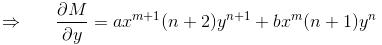∵  The equation is exact now,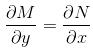Comparing coefficients we get,

a(n + 2) = b(m + 2)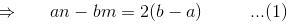and    b(n +1) = a(m +1)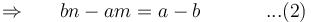abn – b2m = 2(b – a)b          ...(3)
abn – a2m = (a – b)a            ...(4)
Solving (3) and (4), we get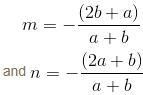The correct answer is: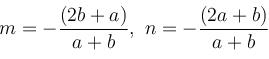QUESTION: 5

The slope of the tangent at the point (xy) to a curve passing through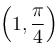is given by,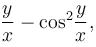then the equation of the curve is :

Solution:

We know that the slope is given by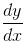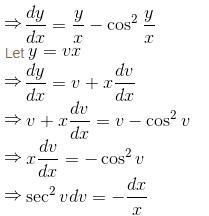Integrating both sides,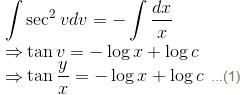Given that the curve passes through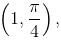so it will satisfy the equation of the curve.
Putting x = 1 and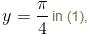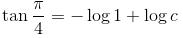1 = log c
⇒ c = e
Putting c = e in (1),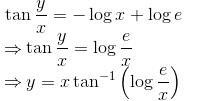is the required curve.

The correct answer is: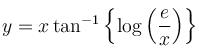QUESTION: 6

The general solution of the equation, (D2 - 6D + 8)y = (e2x - 1)2 + sin3x will be :

Solution:

The auxiliary equation is m2 – 6m + 8 = 0 or (m – 4)(m – 2) =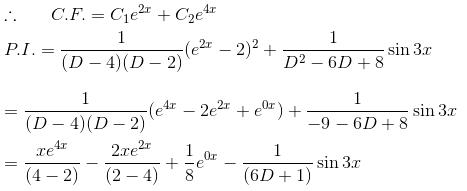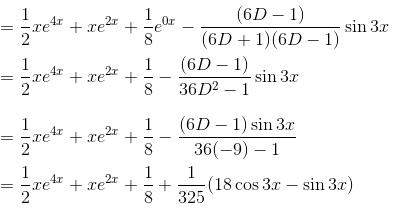Hence the required solution is y = C.F. + P.I.

The correct answer is: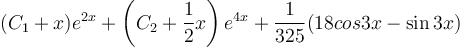QUESTION: 7

If (x, y) is the solution to the system of equations,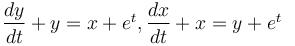then x is equal to :

Solution:

The given equation can be written as
(D – 1)x + (2D + 1)y = 0          ...(1)
2(D + 1)x + (D + 1)y = 3et     ...(2)

Multiplying (1) by 2(D + 1), and (2) by (D – 1) and subtracting,
{2(D + 1)(2D + 1) – (D2 – 1)}y = –3(D – 1)et
= –3 (–et – et) = 6et

or (D2 + 2D + 1)y = 2et          ...(3)
C.F. = (a + bt)et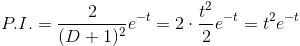The solution of (3) is y = (a + bt + t2)et
The correct answer is: (a + bt)et + tet

QUESTION: 8

The curve orthogonal to the curve x2 + 2xy – y2 = k would be :

Solution:

The given curve is,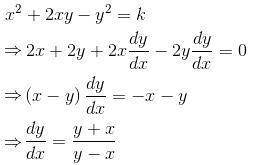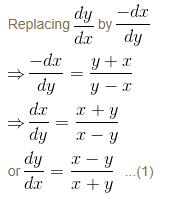which is a homogeneous equation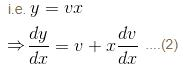from (1) and (2)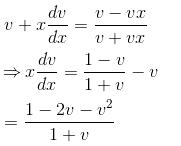Integrating  both sides,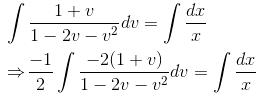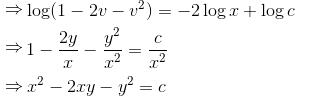The correct answer is: x2 – 2xy – y2 = k

QUESTION: 9

The particular integral of the differential equation,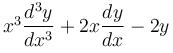= x2 log x + 3x is

Solution:

This is a homogeneous linear equation.

Putting z = log x ⇔ x = ez, the given equation becomes

[D (D – 1) (D – 2) + 2D – 2] = ze2z + 3ez

or    (D3 – 3D2 + 4D – 2) = ze2z + 3z2

The auxiliary equation is m3 – 3m2 + 4m – 2 = 0

⇒  (m – 1) (m2 – 2m + 2) = 0
⇒ m =1, 1 ± i
∴   C.F.
C1ez + ez (C2 cos z + C3 sin z)
x[C1 + C2 cos (log x) + C3 sin (log x)]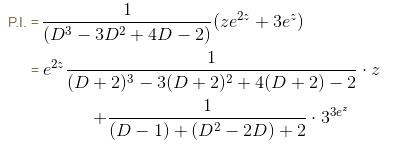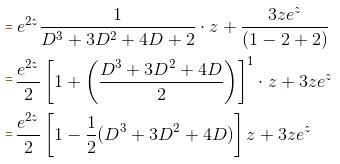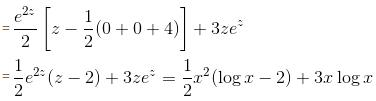The correct answer is: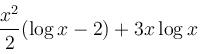QUESTION: 10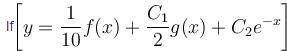is the solution of the differential equation, D2y + 1 (1 - cot x) Dy - y cot x = sin2 x then

Solution:

We have P = 1 – cot xQ = –cot x and so 1 – P + Q = 0.

Thus ex is an integral of D2y + (1 – cot xDy – y cot x = 0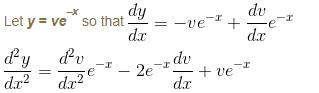The given equation now becomes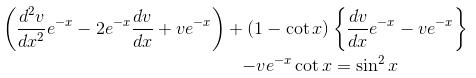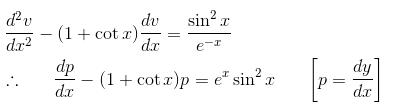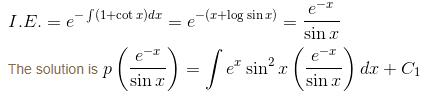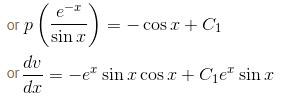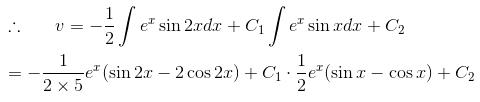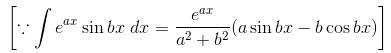Hence,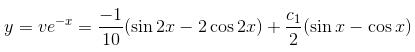is the required solution.

The correct answer is: f(x) = 2 cos 2x – sin 2x and g(x) = sin x – cos x